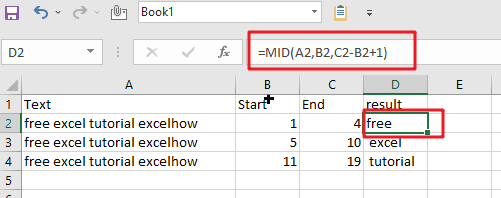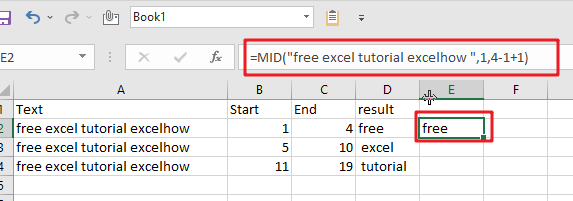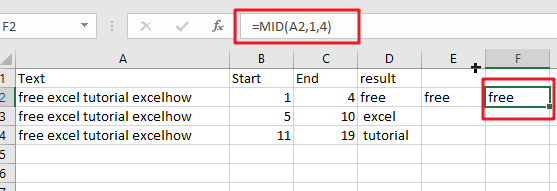# Extract substring In Excel

This post will guide you how to use Excel’s MID function is a quick and easy way to extract pieces from your text. Use the Excel formula to extract a substring with MID.

Note: If you want to extract just a single character at any given time, use the “start” and end positions as your literal start/endpoints. However, if you know how many characters there will be in total (e.g., less than 10), then instead of plugging those numbers into our formula directly. They can easily become calculated values by dividing each side differently!

In the above example, we are going to extract text from a range of cells based on certain criteria. The arguments of our three-argument functions include

1) A text string

2) Starting cell number in B2 with linefeeds replaced by spaces (to make it easier for you).

3) The amount that needs to be removed before reaching C2, where we’ll find out how many characters were actually enclosed between those two points inclusive.

Each time round, these calculations happen, subtracting one point until there’s nothing left but space or a negative #.

Note: Ensure that you have the Text to Columns feature enabled in Excel before attempting this formula; otherwise, it won’t work!

=MID(A2,B2,C2-B2+1)=MID(“free excel tutorial excelhow “,1,4-1+1)=MID(A2,1,4)## Extract Substrings Functions

There are three main extracting substrings functions that are Excel provides.

=MID(Text,start,chars) // extract from middle

=LEFT(Text,chars) // extract from left

=RIGHT(Text,chars) // extract from right

With This Formula Find Start And End Positions:

To handle what start and end positions are, consider the following example.

=MID(B5&C5&D5,”red”,2) // extracts “ed”

The start position of 2 is after the 3rd character, i.e., “r”. Therefore, the number of characters you wish to extract must begin from the start position.

There is no use of Start and end points in the formula because we already know how many characters we want to extract.

### Related Functions

• Excel MID function
The Excel MID function returns a substring from a text string at the position that you specify.The syntax of the MID function is as below:= MID (text, start_num, num_chars)…
• Excel LEFT function
The Excel LEFT function returns a substring (a specified number of the characters) from a text string, starting from the leftmost character.The LEFT function is a build-in function in Microsoft Excel and it is categorized as a Text Function.The syntax of the LEFT function is as below:= LEFT(text,[num_chars])…
• Excel RIGHT function
The Excel RIGHT function returns a substring (a specified number of the characters) from a text string, starting from the rightmost character.The syntax of the RIGHT function is as below:= RIGHT (text,[num_chars])…
Related Posts

How to Split Cells by the First Space in Texts in Excel

Sometimes we may use space to separate texts to different groups in one cell, if we want to split this cell to multiple columns refer to space, we can implement this via ‘Text to Columns’ feature. But is there any ...

How to Convert Uppercase to Lowercase Except the First Letter in Excel

In excel, words can be entered in uppercase, lowercase or mixed. If we want to convert all uppercase to lowercase (except the first letter in some situations), we can use formula with some letter convert related functions to convert them ...

How to Convert Text to Time in Excel

This post will guide you how to convert time string to time in Excel. Assuming that you have a list of text string in your worksheet, and you wish to convert these time string to standard time format, how to ...

How to Remove Last or Trailing Commas in Excel

This post will guide you how to remove trailing commas from cell in Excel. How do I remove comma character at the end of a cell with a formula in Excel 2013/2016. Remove Trailing Commas if you want to remove ...

How to Convert mmddyy to Date in Excel

This post will guide you how to convert non-standard date formats or text to a standard date in Excel. If you have a date with mmddyy format, and you want to change it as the normal date format as mm/dd/yyyy ...

How to Convert Military Time to Standard Time in Excel

This post will guide you how to convert military time to standard time with a formula in Excel. How do I convert from military time 17:29:30 to regular time 5:29:30PM in Excel.  How do I Convert Standard time to military ...

How to Sort Data by Last Character in Excel

This post will guide you how to sort cells by last character in Excel. How do I sort data by the last character with a formula in Excel. How to sort cells based on the last character with User Defined ...

How to Remove the First/Last Word from Text string in Cell

This post will guide you how to remove the first and the last word from a text string in cells in Excel. How do I use a formula to remove first and last word of a text string in Excel. ...

How to Remove Prefix and Suffix in Given Cells in Excel

This post will guide you how to remove suffix form a range of cells in Excel. How do I remove prefix from text string in cells with a formula in Excel. Remove Prefix of Cells Remove Suffix of Cells Assuming ...